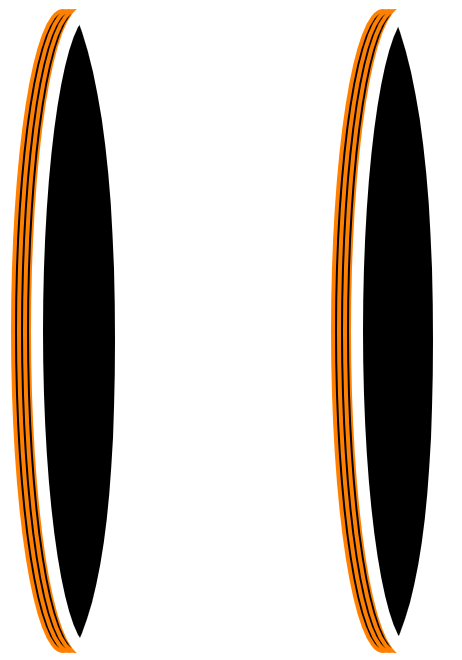# Helmholtz CoilA Helmholtz Coil is a set-up of coils, normally two per axis, that creates a near uniform magnetic field between them. The basic set-up consists of two coils that are separated from each other by a specific distance, for circular coils this is the same as the radius of the coils. Both coils are then powered by an equal current flowing in the same direction.

For Circular Coils:

By setting the distance between the two coils to the radius of the coils, R, the magnetic field uniformity in the center area between the coils is maximized but the field strength at the plane of the coils is around 7% lower. Slightly increasing the distance between the coils improves the uniformity at the coils plane but decreases it in the center. With the distance between the coils of R, the magnetic field, B, in the center is given by the formula bellow:

$$B = {8 \over {5 * \sqrt{5}}} * { {\mu_0 * 10^{-6} * N * I} \over {R} }.$$

Where $\mu_0$ is the permeability of free space, $4 * \pi * 10^{-7}$ Tm/A. In air, the permeability is basically the same so the above equation can be simplified to:

$$B = { {0.8992 * 10^{-6} * N * I} \over {R} }.$$

With B being the generated magnetic field in Tesla, N the number of turns in each coil, I the current through each coil and R the radius of each coil as well as the distance between the two coils.

For Square Coils:

In case of square coils, the formula changes slightly as does the ideal distance between the two coils. With square coils the ideal distance between the two coils is $0.5445 * 2 * a$ (maximized uniformity of the field), with a being half the length of a side of the square coils. With that, the formula that gives the magnetic filed, B, in the center is:

$$B = { 1.01796 } * {{2 * \mu_0 * N * I} \over {\pi * a}}$$

And again, exchanging $\mu_0$ with the permeability of free space, $4 * \pi * 10^{-7}$ Tm/A, the formula is simplified to:

$$B = { {0.8144 * 10^{-6} * N * I} \over {a} }.$$

Bellow are two simple Helmholtz Coil calculators, to aid in designing a helmholtz coil, one for circular coils and one for square coils. Each calculator uses the formulas presented before and assumes that the two coils are separated by the optimal distance to get a uniform field in the center.

The parameters that can be changed, to design the helmholtz coils, are:

• The number of Turns for each coil
• The Current in mA that passes though each coil
• The Radius or Side of the square in cm of each of the coils

It then calculates the magnetic Field in the center of the two coils. It also calculates the Length of wire needed for each coil as well as giving an estimate of what wire Gauge should be used (rule of thumb) and the expected Resistance as well as the power consumption for each coil.